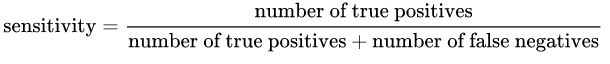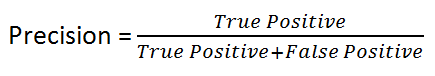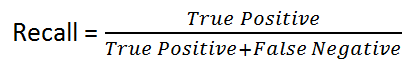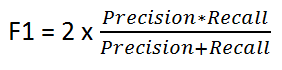2 views

I have the below F1 and AUC scores for 2 different cases

Model 1: Precision: 85.11 Recall: 99.04 F1: 91.55 AUC: 69.94

Model 2: Precision: 85.1 Recall: 98.73 F1: 91.41 AUC: 71.69

The main motive of my problem to predict the positive cases correctly, ie, reduce the False Negative cases (FN). Should I use the F1 score and choose Model 1 or use AUC and choose Model 2. Thanks

by (33.1k points)

## Sensitivity:If the model is 100% sensitive model, that means it didn’t miss any True Positive. Therefore, It predicted every value correct, means no False Negatives. But there is a risk of having a lot of False Positives.

## Specificity:Generally, if we have a 100% specific model, that means it did not miss any True Negative, in other words, there were no False Positives (i.e. negative result that is labeled as positive). But there is a risk of having a lot of False Negatives.

## Precision:Intuitively speaking, if we have a 100% precise model, that means it could catch all True positive but there were NO False Positive.

## Recall:Intuitively speaking, if we have a 100% recall model, that means it didn’t miss any True Positive, in other words, there were no False Negatives (i.e. a positive result that is labeled as negative).

## F1 Score

It's given by the following formula:F1 Score keeps a balance between Precision and Recall. We use it if there is uneven class distribution, as precision and recall may give misleading results.

## AUROC vs F1 Score (Conclusion)

In general, the ROC is used for many different levels of thresholds and thus it has many F score values. F1 score is applicable for any particular point on the ROC curve.

You may think of it as a measure of precision and recall at a particular threshold value whereas AUC is the area under the ROC curve. For F score to be high, both precision and recall should be high.

When you have a data imbalance between positive and negative samples, you should always use F1-score because of ROC averages over all possible thresholds.

Thus, for more study ROC Curve For Machine Learning

If you wish to learn more about Machine learning, visit Machine Learning tutorial and machine learning certification by Intellipaat.